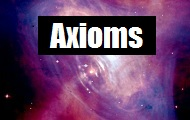Video Samples

 In his Autobiographical Notes in 1949, Einstein wrote of the special theory It is striking that the theory introduces two kinds of physical things, i.e., (1) measuring rods and clocks, and (2) all other things, e.g., the electromagnetic field, the material point, etc.

 A note from Newton's Principles definition #4 in 1687 clearly conflict with Einstein Impressed Force - This force conflicts in the action only; and remains no longer in the body when the action is over.

 In Einstein's second paper on relativity in 1905, he explicitly concludes "Radiation carries inertia between emitting and absorbing bodies". It is important that not only does something receive a "kick" from the momentum of the energy, but the internal inertia (i.e., the inertial mass) of the body is actually increased. (from mathpages.com)

 Frank Wilczek on the "Origins of Mass" (2004 Nobel Prize) Let's see what goes into it (the proton under QCD theory), massless gluons, and massless up and down quarks, and nothing else. You have to agree that that's an explanation of the origin of mass

 Beta Decay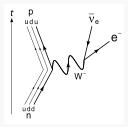The Feynman diagram for beta decay of a neutron into a proton, electron, and electron antineutrino via an intermediate W- boson

Matter and Energy

Matter attracts itself (gravity) and energy repels itself (dark energy). Matter is confined energy and energy travels at the speed of light.
Here we use a fixed grid and fixed timesteps, similar to frames in a movie, to model the particles and forces of matter and energy.
 The Axioms of Animated Physics

# The axioms of Animated Physics

### 1.   Mass is attracted to itself (called gravity)

Newton's laws are used to simulate the motion of objects on the surface of planets, satellites travelling through space and planetary orbits around the sun.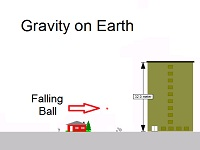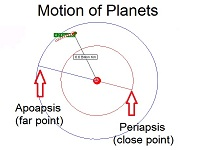The force of attraction between two objects with mass is inversely proportional to the square of the distance between them.

With traditional physics, Newton's force laws allow gravity to be simulated, but start to fail both on the very large scale and on the very small scale. Specifically, the orbit of Mercury cannot be accurately predicted over time with these calculations. In fact, using the inverse square law implies that no two body orbit will have a "precession", yet many are observed. More..

### 2.   Mass is confined energy and energy travels at the speed of light

Einstein's relativity (based on the fixed speed of energy and the relationship between mass and energy) is used to allow for the orbit of Mercury to show its proper precession.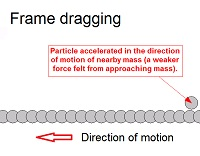The fixed speed of light implies gravitational red shifting, observed elongation of something travelling at close to the speed of light, and different rates of time for observers in motion relative to each other. The fixed speed of energy also predicts frame dragging where mass is accelerated near a fast moving mass in the direction of the moving mass.

Relativistic corrections imply the existence and determine the size of spinning black holes relative to their mass based on the Kerr metric. More..

### 3.   Energy is repelled by itself (called dark energy)

Dark energy (or energy fluctuations at a specific point over time) repels itself in the same way as a wave spreads out over time.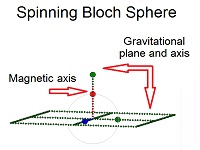There is a value of energy at every point in space (the vacuum is a scalar field) and a connectivity to space where a large fluctuation at one point in space propagates out at the speed of light. Extremely high unstable energy configurations can tear space open and collapse into vortices, specifically the Higgs boson. Different structural vortices together with coupled vortices account for the massive particles.

The distribution and structure of the charge and magnetic field on neutrons, protons and electrons provide the quantum charge, spin and angular momentum values observed. Insight into their properties provides a basis for understanding and modelling the larger scale structure of matter and energy. More..

Animated physics is used here to understand the properties of the real world. Once the forces and objects acting in the grid are quantified in space and time, they can be tested to see if they match reality.